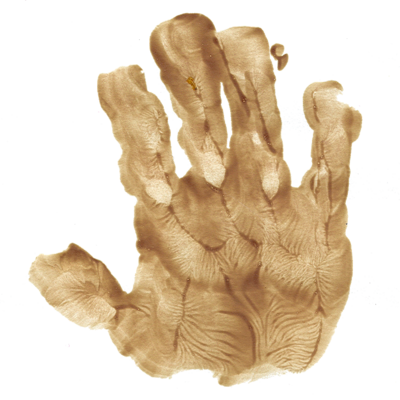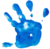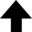We are to admit no more causes of natural things, than such as are both true and sufficient to explain their appearances.Isaac Newton, restating Ockham's idea as the first rule of philosophical reasoning in his great work Principia MathematicaClassical mechanics describes the motion of macroscopic objects, from projectiles to parts of machinery, as well as astronomical objects, such as spacecraft, planets, stars, and galaxies. This page is dedicated to Paul A. Westhaus (1938 – 2018).

## Some Problems in Classical Mechanics

### I. The Double PendulumStart by writing the Cartesian coordinates in terms of polar coordinates:

 $\displaystyle{ x_1 = l_1 \sin \theta_1 = x_1(\theta_1) \text{;} }$ (1.
 $\displaystyle{ y_1 = l_1 \cos \theta_1 = y_1(\theta_1) }$ (1.

for fixed $l_1$. Likewise for the second pendulum:

 $\displaystyle{ x_2 = l_1 \sin \theta_1 + l_2 \sin \theta_2 = x_2(\theta_1, \theta_2) \text{;} }$ (1.
 $\displaystyle{ y_2 = l_1 \cos \theta_1 + l_2 \cos \theta_2 = y_2(\theta_1, \theta_2) }$ (1.

for fixed $l_1$ and $l_2$. Here it should be noted that positive $\theta_2$ is counterclockwise with the choice of positive $l_2 \sin \theta_2$ and $l_2 \cos \theta_2$. The kinetic energy with respect to the inertial reference frame is

 $\displaystyle{ T = \frac{1}{2} m_1 (\dot{x}_1^2 + \dot{y}_1^2) + \frac{1}{2} m_2 (\dot{x}_2^2 + \dot{y}_2^2) \text{,} }$ (1.

where

 $\displaystyle{ \dot{x_1} = \frac{\mathrm{d} x_1}{\mathrm{d} \theta_1} \dot{\theta_1} = l_1 \dot{\theta_1} \cos \theta_1 }$ (1.

and

 $\displaystyle{ \dot{y_1} = \frac{\mathrm{d} y_1}{\mathrm{d} \theta_1} \dot{\theta_1} = -l_1 \dot{\theta_1} \sin \theta_1 \text{.} }$ (1.

Furthermore,

 \displaystyle{ \begin{align*} \dot{x_2} & = \frac{\partial x_2}{\partial \theta_1} \dot{\theta_1} + \frac{\partial x_2}{\partial \theta_2} \dot{\theta_2} + \underbrace{ \frac{\partial x_2}{\partial t} }_\text{= 0} \\ & = l_1 \dot{\theta_1} \cos \theta_1 + l_2 \dot{\theta_2} \cos \theta_2 \text{;} \end{align*} } (1.
 \displaystyle{ \begin{align*} \dot{y_2} & = \frac{\partial y_2}{\partial \theta_1} \dot{\theta_1} + \frac{\partial y_2}{\partial \theta_2} \dot{\theta_2} + \underbrace{ \frac{\partial y_2}{\partial t} }_\text{= 0} \\ & = -l_1 \dot{\theta_1} \sin \theta_1 - l_2 \dot{\theta_2} \sin \theta_2 \text{.} \end{align*} } (1.

The potential energy with respect to the inertial reference frame is

 $\displaystyle{ V = -m_1 g y_1 - m_2 g y_2 \text{.} }$ (1.

The Lagrangian for the double pendulum is therefore

 \displaystyle{ \begin{align*} L & = T - V \\ & = \frac{1}{2} m_1 (l_1 \dot{\theta_1} \cos \theta_1)^2 \\ & + \frac{1}{2} m_1 (-l_1 \dot{\theta_1} \sin \theta_1)^2 \\ & + \frac{1}{2} m_2 (l_1 \dot{\theta_1} \cos \theta_1 + l_2 \dot{\theta_2} \cos \theta_2)^2 \\ & + \frac{1}{2} m_2 (-l_1 \dot{\theta_1} \sin \theta_1 - l_2 \dot{\theta_2} \sin \theta_2)^2 \\ & + m_1 g l_1 \cos \theta_1 \\ & + m_2 g ( l_1 \cos \theta_1 + l_2 \cos \theta_2 ) \text{.} \end{align*} } (1.

Now we'll use Equation (1.11) and the Euler-Lagrange equations in the generalized coordinates $\theta_1$ and $\theta_2$:

 $\displaystyle{ \frac{\partial L}{\partial \theta_1} - \frac{\mathrm{d}}{\mathrm{d} t} \left( \frac{\partial L}{\partial \dot{\theta_1}} \right) = 0 \text{;} }$ (1.
 $\displaystyle{ \frac{\partial L}{\partial \theta_2} - \frac{\mathrm{d}}{\mathrm{d} t} \left( \frac{\partial L}{\partial \dot{\theta_2}} \right) = 0 }$ (1.

to obtain the equations of motion for the double pendulum. These are shown at Hamiltonian Chaos, where they are also solved numerically.

### II. Mass and SpringA small mass $m$ is supported by a vertical spring. When an additional 100 g is attached to the original mass, the system begins to oscillate at a frequency of 0.500 Hz. When the oscillations die out, the spring is found to have increased in length by 10.0 cm. Find $m$.

With just mass $m$ attached to the spring:

 $\displaystyle{ \sum F_y = k y_0 - m g = 0 \text{,} }$ (2.

where $k$ is the spring constant, $y_0$ is the initial compression of the spring, and $g$ is the local acceleration due to gravity. This gives $k = m g / y_0$ as the spring constant. After attaching mass $m'$, we get a reworked but equivalent expression for the spring constant:

 $\displaystyle{ k = \frac{(m + m') g}{y_0 + \Delta y} \text{,} }$ (2.

where $y_0$ is an unknown just as $m$ (our objective) is, and $\Delta y$ is the extra length of the spring after the oscillations die out. The reader should recall that the period of a mass and spring for small oscillations is given by $T = 2 \pi \sqrt{m / k}$. For this situation,

 $\displaystyle{ T = 2 \pi \sqrt{ \frac{m + m'}{k} } \text{,} }$ (2.

or

 $\displaystyle{ \frac{1}{T} \equiv f = \frac{1}{2 \pi} \sqrt{ \frac{k}{m + m'} } \text{.} }$ (2.

Now the frequency $f$ of the oscillations finally makes its appearance.

At this point we should revisit Equation (2.2):

 $\displaystyle{ \frac{k}{m + m'} = \frac{g}{y_0 + \Delta y} \text{,} }$ (2.

with constants in the numerators. We can introduce the frequency in a similar way using Equation (2.4):

 $\displaystyle{ \frac{k}{m + m'} = 4 \pi^2 f^2 \text{.} }$ (2.

Now combine Equations (2.5) and (2.6) to get

 $\displaystyle{ 4 \pi^2 f^2 = \frac{g}{y_0 + \Delta y} \text{,} }$ (2.

where solving for $y_0$ gives 0.893 m using $f$ = 0.500 Hz and $\Delta y$ = 0.100 m. Let's now leverage the spring constant $k$ to write

 $\displaystyle{ \frac{m g}{y_0} = \frac{(m + m') g}{y_0 + \Delta y} \text{.} }$ (2.

Solving Equation (2.8) for the original mass gives:

 $\displaystyle{ m = m' \frac{y_0}{\Delta y} = \boxed{ 893 \text{ g.} } }$ (2.

### III. Deep-Water WavesLet's start by examining a constituent of a water wave—a single water molecule—and then generalize to a whole wave. From the diagram we have a circle (which parameterizes harmonic motion) with radius $A$ and circumference $2 \pi A$. Invoking distance = rate × time, this leads to $v_\text{w} = 2 \pi A / T$, with $T$ equal to the rotational period of the circle. Now connect this to wavelength by recalling $T = 1 / f$ and $v = f \lambda$ to give $v_\text{w} = 2 \pi A v / \lambda$.

Referring to the diagram again, the velocities relative to $\mathbf{v}$ are

 $\displaystyle{ v_\text{t} = v - v_\text{w} = v \left( 1 - \frac{2 \pi A}{\lambda} \right) }$ (3.

for a point at the top of the circle, and

 $\displaystyle{ v_\text{b} = v + v_\text{w} = v \left( 1 + \frac{2 \pi A}{\lambda} \right) }$ (3.

for a point at the bottom of the circle.

At this point we will invoke Bernoulli's equation, which is conservation of energy applied to a dynamic fluid:

 $\displaystyle{ P_\text{t} + \frac{1}{2} \rho v_\text{t}^2 + \rho g y_\text{t} = P_\text{b} + \frac{1}{2} \rho v_\text{b}^2 + \rho g y_\text{b} \text{.} }$ (3.

In Equation (3.3) we will consider a small "hoop", or $P_\text{t} \simeq P_\text{b} \simeq$ atmospheric pressure. After some algebraic wizardry, we can approximate the speed of a deep-water wave:

 $\displaystyle{ \boxed{ v = \sqrt{\frac{g \lambda}{2 \pi}} \text{.} } }$ (3.